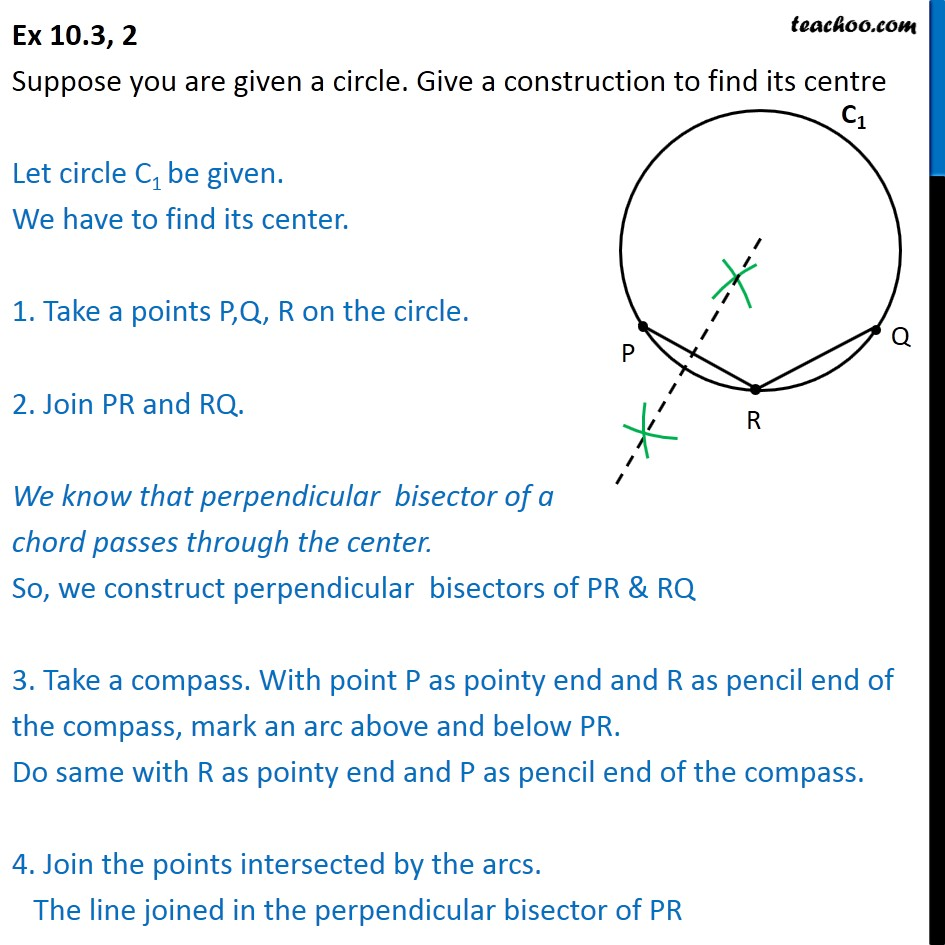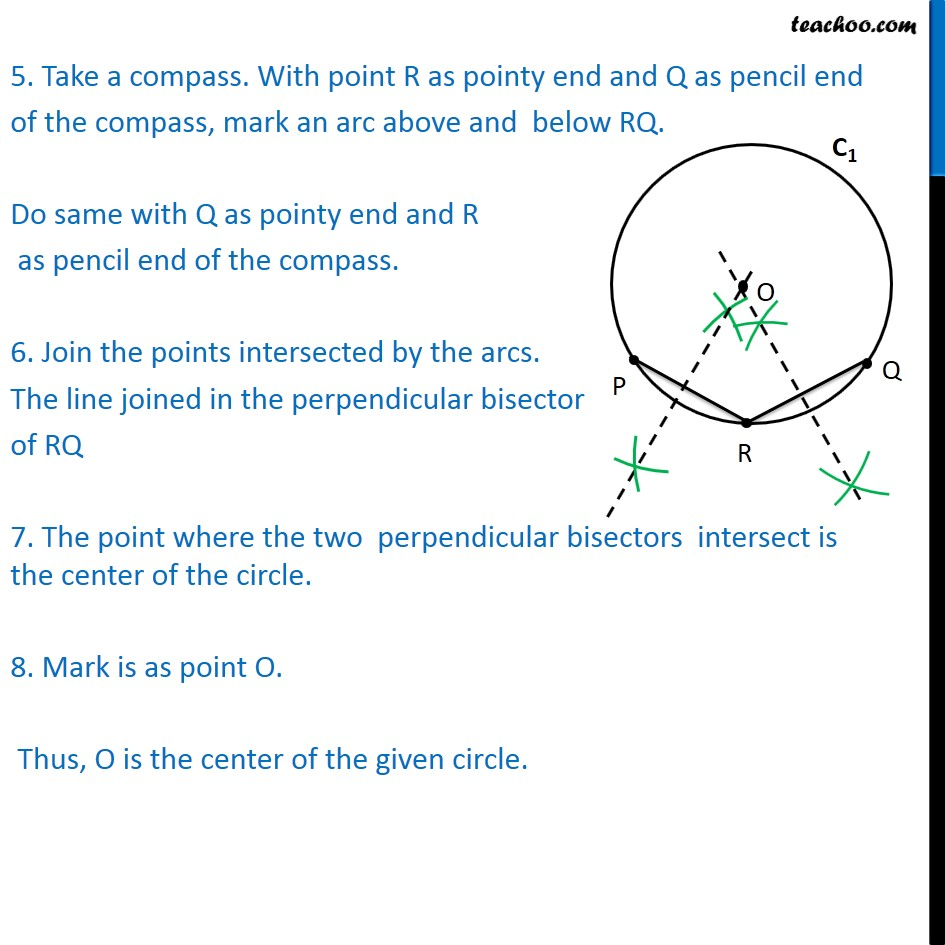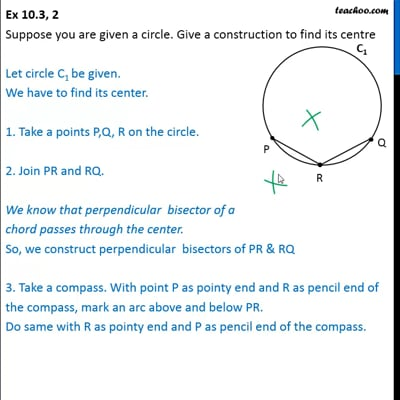Ex 10.3

Chapter 10 Class 9 Circles
Serial order wiseThis video is only available for Teachoo black users

Get live Maths 1-on-1 Classs - Class 6 to 12

### Transcript

Ex 10.3, 2 Suppose you are given a circle. Give a construction to find its centre Let circle C1 be given. We have to find its center. 1. Take a points P,Q, R on the circle. 2. Join PR and RQ. We know that perpendicular bisector of a chord passes through the center. So, we construct perpendicular bisectors of PR & RQ 3. Take a compass. With point P as pointy end and R as pencil end of the compass, mark an arc above and below PR. Do same with R as pointy end and P as pencil end of the compass. 4. Join the points intersected by the arcs. The line joined in the perpendicular bisector of PR 5. Take a compass. With point R as pointy end and Q as pencil end of the compass, mark an arc above and below RQ. Do same with Q as pointy end and R as pencil end of the compass. 6. Join the points intersected by the arcs. The line joined in the perpendicular bisector of RQ 7. The point where the two perpendicular bisectors intersect is the center of the circle. 8. Mark is as point O. Thus, O is the center of the given circle.